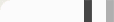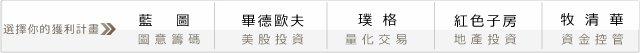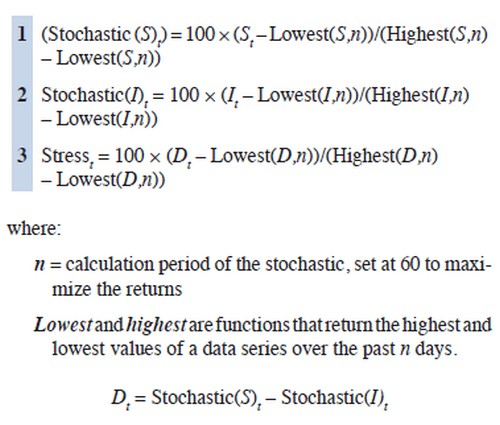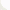## 程式交易新手村－如何撰寫壓力指標？

#### 考夫曼的壓力指標Range1 = Highest( High, N ) - Lowest( Low, N ) ;
Stoch1 = ( Close - Lowest( Low, N ) ) / Range1 ;
Range1這個變數是用來儲存過去N根K棒的最高點減去最低點所計算出的距離，Stoch1則是計算隨機指標的寫法，應該不算太難。

Range2 = Highest( High of Data2, N ) - Lowest( Low of Data2, N ) ;
Stoch2 = ( Close of Data2 - Lowest( Low of Data2, N ) ) / Range2 ;

Diff = Stoch1 - Stoch2;
Range3 = Highest( Diff, N ) - Lowest( Diff, N ) ;
if Range3 ＜＞ 0 then
StressValue = 100 * ( Diff - Lowest( Diff, N ) ) / Range3 ;

StressValue = 50 ;
Stoch1 = 50 ;
Stoch2 = 50 ;
Range1 = Highest( High, N ) - Lowest( Low, N ) ;
Range2 = Highest( High of Data2, N ) - Lowest( Low of Data2, N ) ;

if Range1 ＜＞ 0 and Range2 ＜＞ 0 then begin

Stoch1 = ( Close - Lowest( Low, N ) ) / Range1 ;
Stoch2 = ( Close of Data2 - Lowest( Low of Data2, N ) ) / Range2 ;
Diff = Stoch1 - Stoch2;
Range3 = Highest( Diff, N ) - Lowest( Diff, N ) ;
if Range3 ＜＞ 0 then
StressValue = 100 * ( Diff - Lowest( Diff, N ) ) / Range3 ;

end ;

#### 如何把線畫出來？

Plot1( StressValue, "Stress" ) ;
Plot2( Stoch1 * 100, "D1 Stoch" ) ;
Plot3( Stoch2 * 100, "D2 Stoch" ) ;
Plot4( OBLevel, "OverBought" ) ;
Plot5( OSLevel, "OverSold" ) ;
Plot6( NormalLevel, "Normal" ) ;

#### 可以回頭設定參數與變數了

Inputs:
N( 60 ),
OBLevel( 90 ),
OSLevel( 10 ),
NormalLevel( 50 ) ;

Variables:
Stoch1( 0 ),
Stoch2( 0 ),
Diff( 0 ),
Range1( 0 ),
Range2( 0 ),
StressValue( 0 ) ;

#### 0 意見: